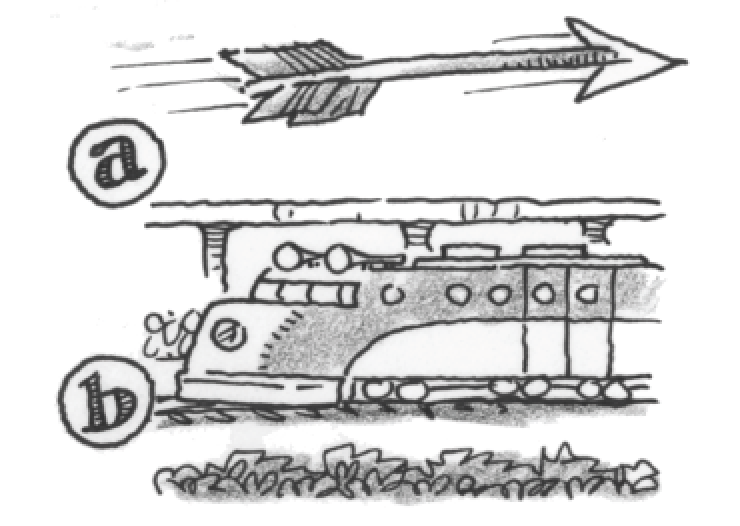### Home > APCALC > Chapter 4 > Lesson 4.2.3 > Problem4-76

4-76.

Compare how distance and velocity are related with these two scenarios:

1. As an arrow flies through the air, the distance it has traveled in feet at time $t$ is given by $s ( t ) = 4 \sqrt { t }$. Without your calculator, determine the velocity, $s^\prime\left(t\right)$, at times $t = 1, 4,$ and $16$ seconds. Explain what concepts of calculus you applied in order to solve this problem.

Three big concepts to choose from are limits, derivatives and integrals.

2. As a train travels past a station, its velocity, measured in miles per hour, is $v\left(t\right) = 9t + 32$. If the train is directly across from the station when $t = 0$, determine the position of the train at $t = 1$ hour. Explain what concepts of calculus you applied in order to solve this problem.

Refer to hint in part (a).

3. Parts (a) and (b) both involve distance and velocity. However, each required a different method or approach. Describe the relationship between distance and velocity, as well as the derivative and area under a curve.

Think back to the Freeway Fatality problem 1-1.